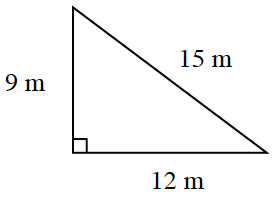### Home > MC2 > Chapter 4 > Lesson 4.3.4 > Problem4-111

4-111.

Sketch the triangle below. Then redraw it with sides that are $\frac { 1 } { 3 }$ as long as the sides of the original.1. Calculate the perimeters of both triangles.

The perimeter is the sum of all the side lengths. In order to find the perimeter of the new triangle, you must multiply each side by one third before adding the side lengths together.

Original triangle: $36$ m
New triangle: $12$ m

2. Calculate the areas of both triangles.

What is the area formula for a triangle?

$\text{Area }=\frac{1}{2}(b)(h)$

3. What is the relationship between the perimeters of the triangles?

Compare the original perimeter ($36$ m) with the new perimeter ($12$ m).
Is there anything the two perimeters have in common?

The perimeter of the original triangle is 3 times the new triangle.